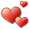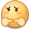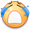# 雅乐网

## Modularity的计算方法——社团检测中模块度计算公式详解

Modularity，中文称为模块度，是 Community Detection（社区发现/社团检测） 中用来衡量社区划分质量的一种方法。要理解Modularity，我们先来看社团和社团检测的概念。

### 社团检测### 公式中用到的字母

#### 和图相关的

n 表示图的结点个数，这里n=14（结点编号0到13）

m 表示图中边的个数，这里m=23（上图中共23条边）

k 表示图中结点的度（degree），无向图中一个结点的度就是该结点连出去的边的条数。显然图中14个结点都有自己的度，我们用$$k_i$$来表示结点i的度。例如：$$k_0 = 3, k_5 = 4, k_6 = 2$$。

A 表示图的邻接矩阵。邻接矩阵A，$$A_{ij} = 1$$表示 结点v 和 结点w 之间有一条边， $$A_{ij} = 0$$表示 结点i 和 结点j 之间没有边。无向图的邻接矩阵是对称的，即$$A_{ij} = A_{ji}$$。特别的，这里结点自己和自己的连接是0，即$$A_{ii} = 0$$，不考虑结点自己和自己的连边。

### Modularity第一版

Newman 在2003年的论文 “Finding and evaluating community structure in networks” 中首次提出了modularity的定义，在论文中用来度量自己的社团检测算法的好坏。

Consider a particular division of a network into k communities. Let us define a k×k symmetric matrix e whose element $$e_{ij}$$ is the fraction of all edges in the network that link vertices in community i to vertices in community j .As discussed in , it is crucial to make sure each edge is counted only once in the matrix eij—the same edge should not appear both above and below the diagonal. Alternatively, an edge linking communities i and j can be split, half-and-half, between the ij and ji elements, which has the advantage of making the matrix symmetric. Either way, there are a number of factors of 2 in the calculation that must be watched carefully, lest they escape one’s attention and make mischief.

$$e_{32} = \frac{2}{23}， e_{23} = \frac{0}{23}$$ 或者 $$e_{32} = \frac{0}{23}， e_{23} = \frac{2}{23}$$ 或者$$e_{32} = \frac{1}{23} ， e_{23} = \frac{1}{23}$$

 1 2 3 1 $$\frac{5}{23}$$ $$\frac{1}{2} \times \frac{1}{23}$$ $$\frac{1}{2} \times \frac{0}{23}$$ 2 $$\frac{1}{2} \times \frac{1}{23}$$ $$\frac{7}{23}$$ $$\frac{1}{2} \times \frac{2}{23}$$ 3 $$\frac{1}{2} \times \frac{0}{23}$$ $$\frac{1}{2} \times \frac{2}{23}$$ $$\frac{8}{23}$$

So we further define the row (or column) sums $$a_i = \sum_{j}{e_{ij}}$$ , which represent the fraction of edges that connect to vertices in community i.

$$a_i = \sum_{j}{e_{ij}}$$，它表示连接到 社团i 中的边的端点数（相当于社团中所有点的度相加） 占总端点数（2m） 的比例 ，即

$$a_i = \frac{k_{C_i}}{2m}$$

$$k_{C_i}$$表示社团i内部所有点的度数之和。

 a 1 $$\frac{5}{23} + \frac{1}{2} \times \frac{1}{23} + \frac{1}{2} \times \frac{0}{23}$$ = $$\frac{11}{46}$$ 2 $$\frac{1}{2} \times \frac{1}{23} + \frac{7}{23} + \frac{1}{2} \times \frac{2}{23}$$ = $$\frac{17}{46}$$ 3 $$\frac{1}{2} \times \frac{0}{23} + \frac{1}{2} \times \frac{2}{23} + \frac{8}{23}$$ = $$\frac{18}{46}$$

In a network in which edges fall between vertices without regard for the communities they belong to, we would have $$e_{ij} = a_ia_j$$.

Thus we can define a modularity measure by

$$Q = \sum_i{(e_{ii} – a_i^2)} = Tr e – ||e^2||$$

where ||x|| indicates the sum of the elements of the matrix x.

$$Q = \sum_i{(e_{ii} – a_i^2)} = \sum_i{e_{ii}} – \sum_i{ a_i^2}$$

$$Q = \sum_i{(\frac{e_i}{m} – (\frac{k_{C_i}}{2m})^2)}$$

$$Q = \frac{5}{23} + \frac{7}{23} + \frac{8}{23} – (\frac{11}{46})^2 – (\frac{17}{46})^2 – (\frac{18}{46})^2 = \frac{553}{1058} = 0.52268431$$

$$Q = \sum_i{(e_{ii} – a_i^2)} = \sum_i{e_{ii}} – \sum_i{ a_i^2} = Tr e – ||e^2||$$

$$\sum_i{ ( \sum_{j}{e_{ij}} )^2} = ||e^2||$$

$$\sum_i{ a_i^2} = (\frac{5}{23} + \frac{1}{46} + 0)^2 + (\frac{1}{46} + \frac{7}{23} + \frac{1}{23})^2 + (0 + \frac{1}{23} + \frac{8}{23})^2$$

$$e^2 = \begin{pmatrix} \frac{5}{23} & \frac{1}{46} & 0 \\ \frac{1}{46} & \frac{7}{23} & \frac{1}{23} \\ 0 & \frac{1}{23} & \frac{8}{23} \\ \end{pmatrix} \times \begin{pmatrix} \frac{5}{23} & \frac{1}{46} & 0 \\ \frac{1}{46} & \frac{7}{23} & \frac{1}{23} \\ 0 & \frac{1}{23} & \frac{8}{23} \\ \end{pmatrix} =$$

### Modularity第二版

#### Newman论文中的定义

2006年，Newman 在论文“Modularity and community structure in networks”中重新定义了modularity，目的是为了满足spectral properties。原话为：

Here we take a different approach based on a reformulation of the modularity in terms of the spectral properties of the network of interest.

Suppose our network contains n vertices.

n 表示图的结点个数

For a particular division of the network into two groups let s_i = 1 if vertex i belongs to group 1 and s_i = -1 if it belongs to group 2. Observing that the quantity $$\frac{1}{2} (s_i s_j + 1)$$  is 1 if i and j are in the same group and 0 otherwise

And let the number of edges between vertices i and j be $$A_{ij}$$, which will normally be 0 or 1, although larger values are possible in networks where multiple edges are allowed.(The quantities $$A_{ij}$$ are the elements of the so-called adjacency matrix.)

1. 无向图的邻接矩阵是对称的，即$$A_{ij} = A_{ji}$$。因此，如果把矩阵A的所有元素相加，得到的值是图中边的数目的2倍：$$2m = \sum{A_{ij}}$$。

2. 自己和自己的连接在矩阵中是0，即$$A_{ii} = 0$$。

At the same time, the expected number of edges between vertices i and j if edges are placed at random is $$\frac{k_ik_j}{2m}$$, where $$k_i$$ and $$k_j$$ are the degrees of the vertices and $$m = \frac{1}{2} \sum_i{k_i}$$ is the total number of edges in the network.

Thus the modularity Q is given by the sum of $$A_{ij} – \frac{k_ik_j}{2m}$$   over all pairs of vertices i, j that fall in the same group.

$$A_{ij} – \frac{k_ik_j}{2m}$$ 表示的是结点i和结点j之间的连边数，减去 随机情况下 结点i和结点j之间的期望连边数。（关于随机情况的讨论在下面）

we can then express the modularity as

$$Q = \frac{1}{4m} \sum_{ij}{(A_{ij} – \frac{k_ik_j}{2m})(s_is_j+1)}$$

The leading factor of $$\frac{1}{4m}$$ is merely conventional: it is included for compatibility with the previous definition of modularity (17).

Q计算公式前面的$$\frac{1}{4m}$$仅仅是为了和之前第一版的modularity计算公式相兼容。

$$Q = \frac{1}{2m} \sum_{ij}{(A_{ij} – \frac{k_ik_j}{2m})\frac{(s_is_j+1)}{2}}$$

$$Q = \frac{1}{2m} \sum_{ij}{(A_{ij} – \frac{k_ik_j}{2m}) \delta(i, j)}$$

$$Q = \frac{1}{2m} \sum_{i,j在同一社团}{(A_{ij} – \frac{k_ik_j}{2m}) }$$

$$A_{ij}$$ 表示 结点i 和 结点j 之间边的数目

$$\frac{k_ik_j}{2m}$$ 表示 随机放置边的情况下，结点i和结点j 之间边数的期望值

$$\sum_{i,j}{A_{ij}}$$ 就表示 社团内部实际的 边 的数目 的2倍（2倍是因为ij和ji会计算2次）

$$\sum_{i,j}{\frac{k_ik_j}{2m}}$$ 就表示 随机放置边的情况下，社团内部边数的期望值 的2倍（2倍是因为ij和ji会计算2次）

$$\frac{1}{2m} \sum_{i,j}{A_{ij}}$$ 就表示 社团内部实际的边数的比例

$$\frac{1}{2m} \sum_{i,j}{\frac{k_ik_j}{2m}}$$就表示 随机情况下社团内部期望的边数的比例

#### 期望边数$$\frac{k_ik_j}{2m}$$的来源

1.每个结点度不变，最终总边数也不会变。因此随机连边后，图中的边数期望值等于原来真实图中的边数

$$\sum_{i,j}{P_{ij}} = \sum_{i,j}{A_{ij}} = 2m$$

2.对每个节点来说，它的度也不会变，就有

$$\sum_{j}{P_{ij}} = k_i$$

$$P_{ij} = f(k_i)f(k_j)$$

$$\sum_{j}{P_{ij}} = \sum_{j}{f(k_i)f(k_j)} = f(k_i) \sum_{j}{f(k_j)}$$

$$f(k_i) \sum_{j}{f(k_j)} = k_i$$

$$f(k_i) = C k_i$$

C是常量。代入上面性质的第一个式子$$\sum_{i,j}{P_{ij}} = \sum_{i,j}{A_{ij}} = 2m$$ ，有

$$\sum_{i,j}{P_{ij}} = 2m$$

$$\sum_{i,j}{f(k_i)f(k_j)} = 2m$$

$$\sum_{i,j}{C k_i C k_j} = 2m$$

$$C^2 \sum_{ij}{k_ik_j} = 2m$$

$$\sum_{ij}{k_ik_j}$$是可以用m表示的。假设结点编号从1到n，则

$$2m = k_1 + k_2 + … + k_n$$

$$(2m)^2 = (k_1 + k_2 + … + k_n)^2$$

$$(2m)^2 = (k_1 + k_2 + … + k_n)^2 = \sum_{ij}{k_ik_j}$$

$$C^2 (2m)^2 = 2m$$

$$C = \frac{1}{\sqrt{2m}}$$

$$P_{ij} = f(k_i)f(k_j) = C^2 k_i k_j = \frac{k_ik_j}{2m}$$

#### configuration model

configuration model是一种生成图的模型，它已知每个结点的度$$k_i$$，然后随机生成边。$$P_{ij} = \frac{k_ik_j}{2m-1}$$

### 版本二和版本一的关系

$$Q = \sum_i^c{(e_{ii} – a_i^2)} = \sum_i{e_{ii}} – \sum_i{ a_i^2}$$

$$Q = \frac{1}{2m} \sum_{i,j在同一社团}{(A_{ij} – \frac{k_ik_j}{2m}) }$$

$$\sum_i{e_{ii}} – \sum_i{ a_i^2} = \frac{1}{2m} \sum_{i,j在同一社团}{(A_{ij} – \frac{k_ik_j}{2m}) }$$

$$\sum_i^c{e_{ii}} = \frac{1}{2m} \sum_{i,j在同一社团}{(A_{ij} )}$$

$$e_{ii}$$表示的是社团i内部边的比例，求和后就是所有社团内部的边的比例。右边的求和$$\sum{(A_{ij} }$$就等于内部边数的2倍，除以2m也就是社团内部边的比例。这两个相等是容易看出的。

$$\sum_i^c{ a_i^2} = \frac{1}{2m} \sum_{i,j在同一社团}{(\frac{k_ik_j}{2m}) }$$

$$a_i^2$$就是社团i内结点的度数之和的平方 / $$(2m)^2$$

$$右边= \frac{1}{2m} \sum_{i,j在同一社团}{(\frac{k_ik_j}{2m}) } = \frac{1}{(2m)^2} \sum_{i,j在同一社团}{(k_ik_j) }$$

$$Q = \sum_i{e_{ii}} – \sum_i{ a_i^2} = \frac{1}{2m} \sum_{i,j在同一社团}{(A_{ij} – \frac{k_ik_j}{2m}) }$$

### 针对有权图和重叠社团的推广

$$Q = \sum_k{(\frac{e_{kk}}{m} – (\frac{d_k}{2m})^2)}$$

$$e_{kk} = \frac{1}{2} \sum_{i,j \in C_k} \frac{w_{ik} + w_{jk}}{2} A_{ij}$$

$$A$$是图的邻接矩阵，$$A_{ij}$$也就是对应边的权值。

$$e_{k_out} = \sum_{i \in C_k, j \notin C_k} (w_{ik} + (1 – w_{jk})) A_{ij}$$

$$d_k = 2e_{kk} + e_{k_out}$$

### C++代码计算

Modularity计算的C++代码 | 雅乐网

http://www.yalewoo.com/modularity_community_detection.html

### 文章《Modularity的计算方法——社团检测中模块度计算公式详解》共有21条评论：

1.匿名

楼主，那个 e矩阵的每行求和，然后平方，再相加 等于 e矩阵平方再求和 我会证了，你需要不？

•yalewoo 作者

谢谢，我毕业后就没做这方面了，如果你的证明有博客可以贴一下地址哈 可以给大家参考下

•匿名

不好意思，不太会贴。你可以留下你的联系方式，一起交流。

2.羊梨酥

hallo 我想问一下我在用python的networkx中有个comunity的包划分网络后，计算得出的modularity>1,会有这种特殊情况嘛？ 作者大大有没有看到过类似的情况？
代码如下：
part=community.best_partition(G)#用 lovain社区检测算法划分模块
modular=community.modularity(part,G)#计算modularity

我输入的网络是无向带权重图 但是权重是浮点数 不知道会不会影响他的计算结果

•yalewoo 作者

我没有用过networkx哎，可以看一下他里面modularity的文档吧 看有没有说明结果范围

3.TONG WU

请问，矩阵相乘不是对应元素两两相乘吧？

•yalewoo 作者

hi，相乘应该是第一个的第一行点乘第二个的第一列，你是说||e^2||那里吗，前面(a+b+c)^2拆开后也会有别的项应该正好对应矩阵相乘的法则

4.匿名

全网最赞 真的

•yalewoo 作者

谢谢 这是我收到的最开心的赞5.征兆

太棒了，比其他解释都清楚明白！

•yalewoo 作者

谢谢6.匿名

很赞！

•yalewoo 作者谢谢

7.six-archer

如果按照这个算法去算Q的值的话，把每一个点都当成一个群的话算出来不是负的值吗？但是Q的范围不是应该在0-1之间的吗？求大佬解释一下

•yalewoo 作者

维基百科上说 Q的范围是 [−1/2,1) ，具体怎么得出的我也不懂https://en.wikipedia.org/wiki/Modularity_(networks)

•匿名

另外请问一下，这种以Q为判断依据在有向图中是不是不能使用的

•yalewoo 作者

•匿名

当只有两个点且两个点间有一条边时，把两个点各划分为一类好像会得到-0.5，好像没有更小的情况了。
当有很多个团时(n)，且团间没有边时，Q = 1/n+1/n+……+1/n-(1/n)²-(1/n)²-……-(1/n)²=1-1/n，当n趋于无穷时好像可以到1吧。

•匿名

Newman文章中提到 “if the number of within-community edges is no better than random, Q=0″，所以Q应该是0到1.

维基百科引用的那篇文章证明了Q在[-1/2, 1]之间（没看懂），但是也提到，In particular, the modularity of a trivial clustering that places all vertices into a single cluster has a value of 0.

8.HCaffrey

In a network in which edges fall between vertices without regard for the communities they belong to, we would have eij=aiaj

把这个式子代入ai = sum_j(eiej)，式子等价于sum_j(aj) = 1
Meaning it is a random network regarding all of its nodes as individual community

sum_j 表示连加符号，抱歉没能插入公式

9.zy